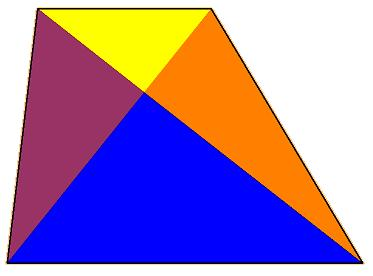#### You may also like### At a Glance

The area of a regular pentagon looks about twice as a big as the pentangle star drawn within it. Is it?### Six Discs

Six circular discs are packed in different-shaped boxes so that the discs touch their neighbours and the sides of the box. Can you put the boxes in order according to the areas of their bases?### Equilateral Areas

ABC and DEF are equilateral triangles of side 3 and 4 respectively. Construct an equilateral triangle whose area is the sum of the area of ABC and DEF.

# Trapezium Four

##### Age 14 to 16Challenge LevelWell done to Dominic from St Paul's School, Tom from Bristol Grammar School, and Margaret in Cornwall who in different ways pointed out that :

When you put together the purple and blue triangles this larger triangle must have the same area as the larger triangle made from orange and blue because they share the same base and have the same height, therefore must match for area. Then as blue is common to both these triangles the bits that are purple and orange must also be equal in area.

Well done Margaret for pointing out that yellow and blue are similar triangles :

So if, looking at the two parallel side lengths, we said that the longer side is $k$ times the shorter then the blue triangle has $k^2$ times the area of yellow because the blue height is $k$ times bigger than the yellow height.

Looking along a diagonal of the trapezium and comparing yellow with purple they have the same "height away from the diagonal" but purple's "base along that diagonal" is $k$ times as big as yellow's base (those similar triangles again) and so purple's area will be $k$ times as big as the area of yellow.

Comparing all four areas : purple and orange will always be equal, yellow will always be smaller than them while blue will always be larger.

The ratio of areas for yellow, purple, orange and blue is $1 : k : k : k^2$.

Well done to Tom and Dominic for pointing out that the only time anything happens other than just two areas equal is on the occasion that the parallel sides are the same length, the diagonals then intersect at their midpoints, the trapezium at that moment becomes a parallelogram, and the four colours occupy equal areas.